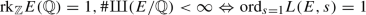# On the soft p-converse to a theorem of Gross–Zagier and Kolyvagin

## Abstract

We give a proof of a soft version of the p-converse to a theorem of Gross–Zagier and Kolyvagin for non-CM elliptic curves with good ordinary reduction at $$p >3$$ under the irreducibility assumption on the residual representation. In particular, no condition on the conductor is imposed. Combining with the known results, we obtain the equivalencefor every elliptic curve E over $$\mathbb {Q}$$.

This is a preview of subscription content, access via your institution.

## Data availability

Data sharing not applicable to this article as no datasets were generated or analysed during the current study.

## References

1. Burungale, A.A., Castella, F., Kim, C.-H.: A proof of Perrin-Riou’s Heegner point main conjecture. Algebra Number Theory 15(10), 1627–1653 (2021)

2. Burungale, A.A., Castella, F., Skinner, C., Tian, Y.: $$p^\infty$$-Selmer groups and rational points on CM elliptic curves. Ann. Math. Québec 46(2), 324–346 (2022)

3. Bertolini, M., Darmon, H., Venerucci, R.: Heegner points and Beilinson-Kato elements: a conjecture of Perrin-Riou. Adv. Math. 398, 108172 (2022)

4. Bump, D., Friedberg, S., Hoffstein, J.: Nonvanishing theorems for $$L$$-functions of modular forms and their derivatives. Invent. Math. 102(3), 543–618 (1990)

5. Büyükboduk, K., Pollack, R., Sasaki, S.: $$p$$-adic Gross–Zagier formula at critical slope and a conjecture of Perrin-Riou, preprint, arXiv:1811.08216

6. Burungale, A.A., Skinner, C., Tian, Y.: Elliptic curves and Beilinson–Kato elements: rank one aspects, preprint

7. Burungale, A.A., Skinner, C., Tian, Y.: The Birch and Swinnerton-Dyer conjecture: a brief survey, Nine mathematical challenges–an elucidation (Providence, RI) (A. Kechris, N. Makarov, D. Ramakrishnan, and X. Zhu, eds.), Proc. Sympos. Pure Math., vol. 104, Amer. Math. Soc., 2021, pp. 11–29

8. Burungale, A.A., Tian, Y.: $$p$$-converse to a theorem of Gross-Zagier, Kolyvagin and Rubin. Invent. Math. 220(1), 211–253 (2020)

9. Büyükboduk, K.: Beilinson–Kato and Beilinson–Flach elements, Coleman–Rubin–Stark classes, Heegner points and a conjecture of Perrin-Riou, Development of Iwasawa Theory – the Centennial of K. Iwasawa’s Birth (Tokyo) (Masato Kurihara, Kenichi Bannai, Tadashi Ochiai, and Takeshi Tsuji, eds.), Adv. Stud. Pure Math., vol. 86, Mathematical Society of Japan, 2020, pp. 141–193

10. Castella, F., Grossi, G., Lee, J., Skinner, C.: On the anticyclotomic Iwasawa theory of rational elliptic curves at Eisenstein primes. Invent. Math. 227, 517–580 (2022)

11. Castella, F., Wan, X.: Perrin-Riou’s main conjecture for elliptic curves at supersingular primes, preprint

12. Gross, B., Zagier, D.: Heegner points and derivatives of $$L$$-series. Invent. Math. 84(2), 225–320 (1986)

13. Kato, K.: $$p$$-adic Hodge theory and values of zeta functions of modular forms. Astérisque 295, 117–290 (2004)

14. Kolyvagin, V.: Euler systems, The Grothendieck Festschrift Volume II (Pierre Cartier, Luc Illusie, Nicholas M. Katz, Gerard Laumon, Yuri Manin, and Kenneth A. Ribet, eds.), Progr. Math., vol. 87, Birkhäuser Boston, 1990, pp. 435–483

15. Kurihara, M.: On the Tate Shafarevich groups over cyclotomic fields of an elliptic curve with supersingular reduction I. Invent. Math. 149, 195–224 (2002)

16. Murty, M.R., Murty, V.K.: Mean values of derivatives of modular $$L$$-series. Ann. Math. 133(3), 447–475 (1991)

17. Perrin-Riou, B.: Fonctions $$L$$$$p$$-adiques d’une courbe elliptique et points rationnels. Ann. Inst. Fourier (Grenoble) 43(4), 945–995 (1993)

18. Rubin, K.: Tate-Shafarevich groups and $$L$$-functions of elliptic curves with complex multiplication. Invent. Math. 89, 527–559 (1987)

19. Rubin, K.: $$p$$-adic variants of the Birch and Swinnerton-Dyer conjecture for elliptic curves with complex multiplication, $$p$$-adic monodromy and the Birch and Swinnerton-Dyer conjecture (Boston, MA, 1991) (Providence, RI) (Barry Mazur and Glenn Stevens, eds.), Contemp. Math., vol. 165, American Mathematical Society, 1994, pp. 71–80

20. Karl, R.: Euler systems and modular elliptic curves, Galois representations in arithmetic algebraic geometry (Durham, 1996) (Anthony Scholl and Richard Taylor, eds.), London Math. Soc. Lecture Note Ser., vol. 254, Cambridge University Press, 1998, pp. 351–367

21. Serre, J.-P.: Quelques applications du théorème de densité de Chebotarev. Publ. Math. Inst. Hautes Études Sci. 54, 323–401 (1981)

22. Serre, J.-P.: Abelian $$\ell$$-adic representations and elliptic curves, Advanced Book Classics, Addison-Wesley Publishing Company, 1989, With the collaboration of Willem Kuyk and John Labute

23. Skinner, C.: A converse to a theorem of Gross, Zagier, and Kolyvagin. Ann. Math. 191(2), 329–354 (2020)

24. Skinner, C., Urban, E.: The Iwasawa main conjectures for $${{\rm GL}}_2$$. Invent. Math. 195(1), 1–277 (2014)

25. Sweeting, N.: Kolyvagin’s conjecture and patched Euler systems in anticyclotomic Iwasawa theory, preprint, arXiv:2012.11771

26. Skinner, C., Zhang, W.: Indivisibility of Heegner points in the multiplicative case, preprint, arXiv:1407.1099

27. Venerucci, R.: Exceptional zero formulae and a conjecture of Perrin-Riou. Invent. Math. 203(3), 923–972 (2016)

28. Venerucci, R.: On the $$p$$-converse of the Kolyvagin-Gross-Zagier theorem. Comment. Math. Helv. 91(3), 397–444 (2016)

29. Wan, X.: The Iwasawa main conjecture for Hilbert modular forms. Forum Math. Sigma 3, e18 (2015)

30. Wan, X.: Heegner point Kolyvagin system and Iwasawa main conjecture. Acta Math. Sin. 37(1), 104–120 (2021)

31. Zhang, W.: Selmer groups and the indivisibility of Heegner points. Camb. J. Math. 2(2), 191–253 (2014)

## Acknowledgements

The discussion with Ashay Burungale and Francesc Castella leads us to improve the main result and the exposition in an earlier version significantly. We deeply thank to both. This research was partially supported by a KIAS Individual Grant (SP054102) via the Center for Mathematical Challenges at Korea Institute for Advanced Study and by the National Research Foundation of Korea(NRF) grant funded by the Korea government(MSIT) (No. 2018R1C1B6007009). We would like to thank the referee for carefully reading our manuscript and for giving constructive and valuable comments.

## Author information

Authors

### Corresponding author

Correspondence to Chan-Ho Kim.

## Ethics declarations

### Conflict of interest

The author has no relevant financial or non-financial interests to disclose.